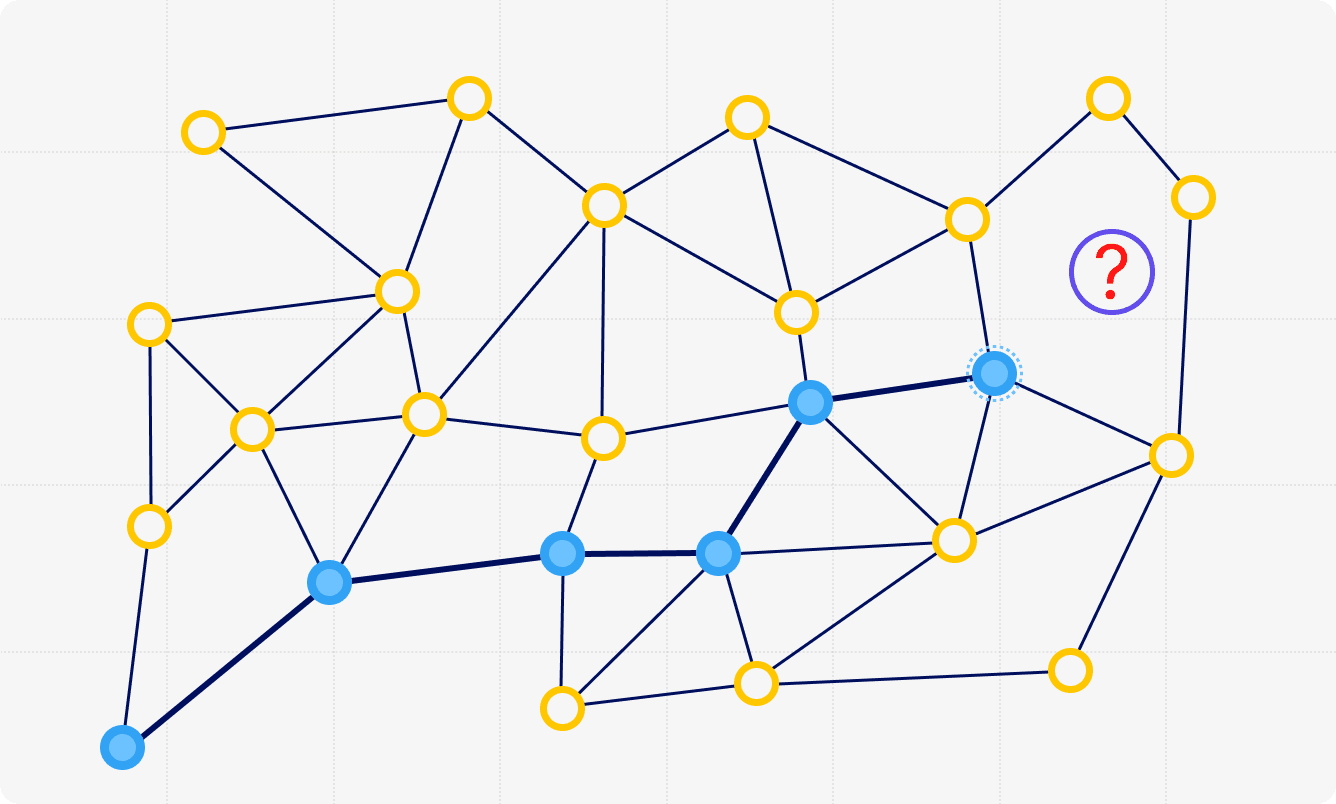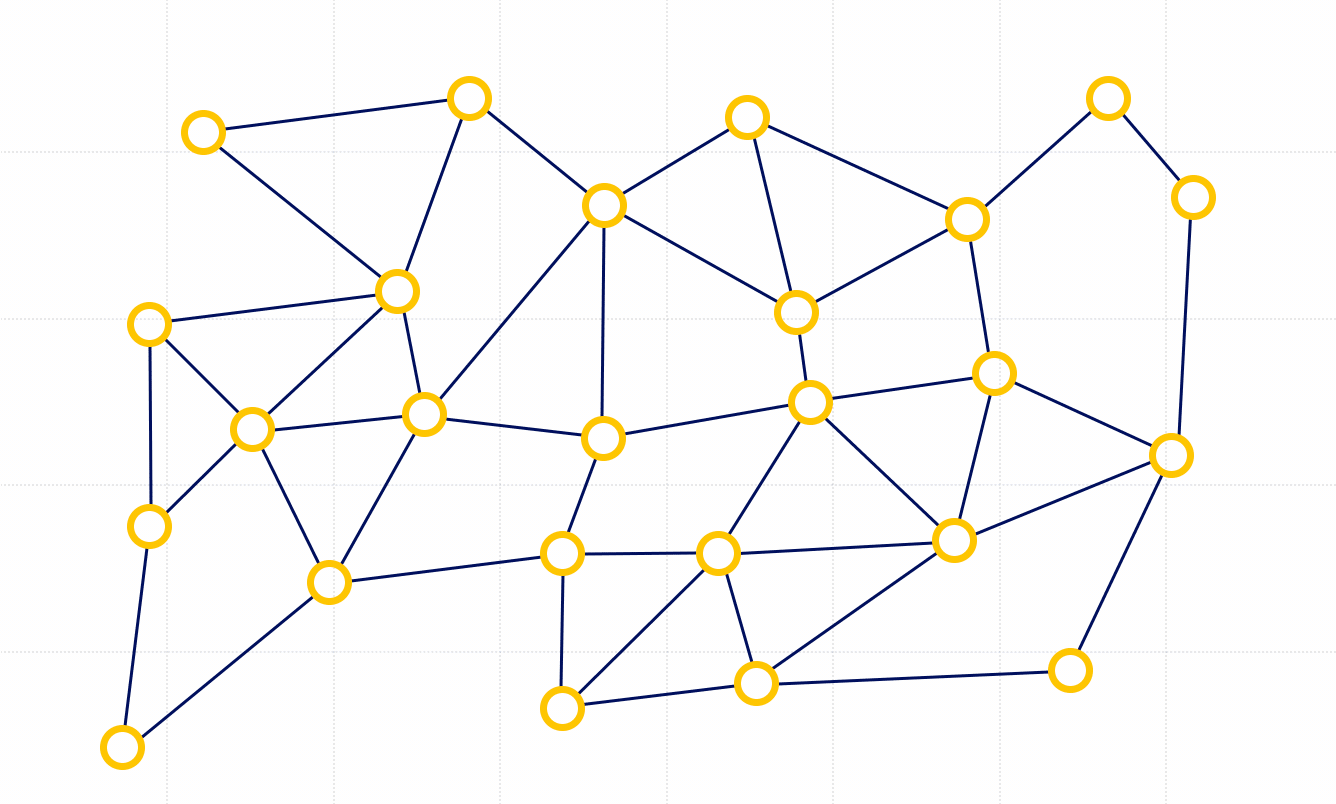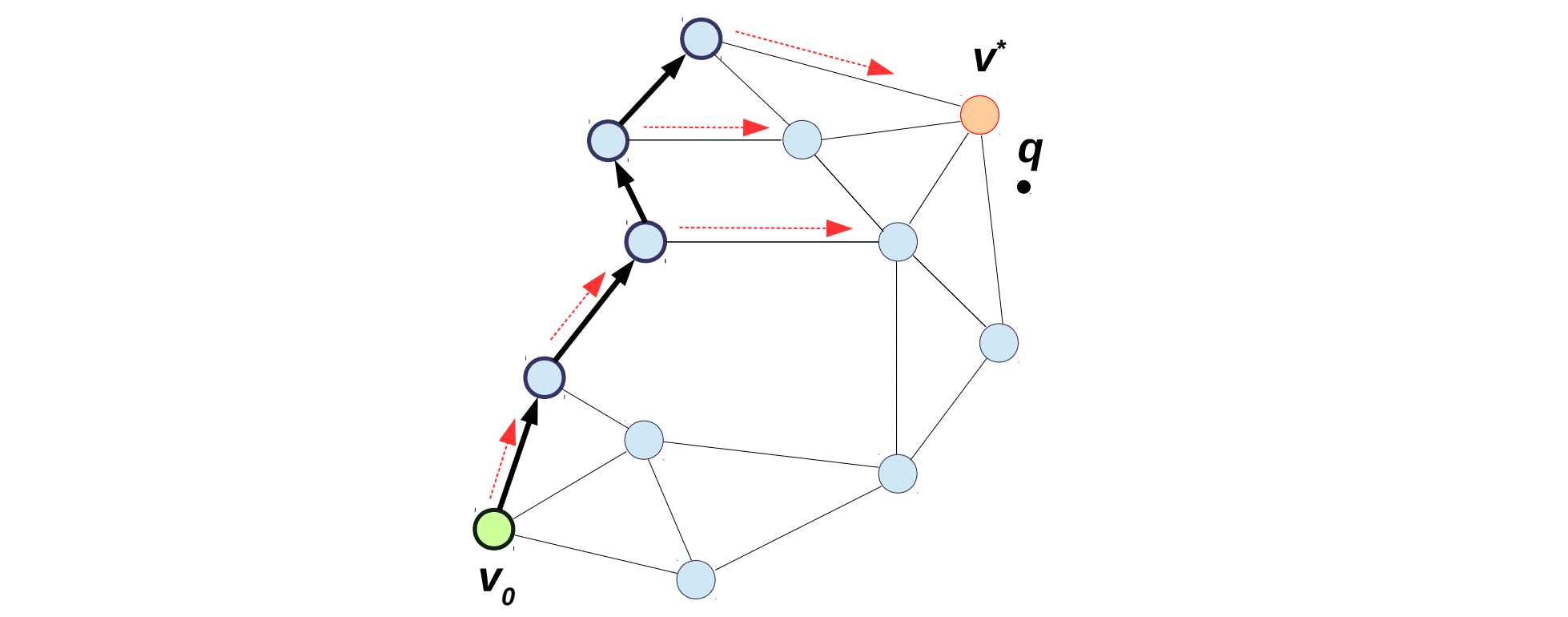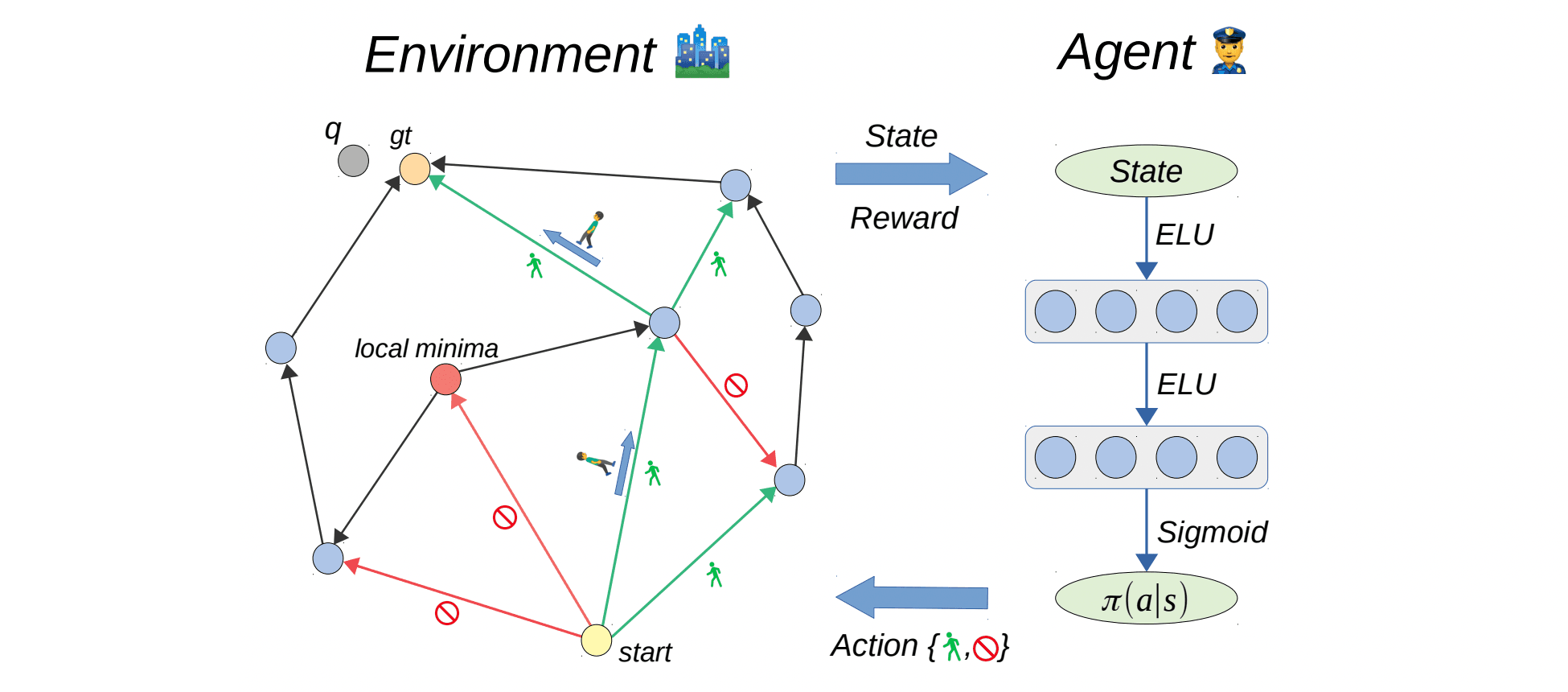# Graph-based nearest neighbor searchNearest Neighbor Search (NNS) is a long-standing problem arising in many machine learning applications, such as recommender services, information retrieval, and others. The goal of the nearest neighbor search is to find the closest element to a given query vector within a database of $n$ elements. Since the sizes of databases in practical tasks are constantly increasing, the scalability of NNS becomes crucial.
Efficient NNS receives much attention from the machine learning community. The problem is well-solved for low-dimensional spaces. However, most practical applications require efficient and accurate search in high-dimensional ones. Due to the curse of dimensionality, for large $d$ the algorithms for exact NNS provide little improvement over a brute-force algorithm. Thus, both researchers and practitioners are interested in approximate solutions which are efficient and quite accurate.
Most approximate NNS algorithms rely on indexing structures. Well-known established indexing structures based on partition trees

, inverted indices

and locality-sensitive hashing (LSH)
have been extensively explored by ML researchers and provide both decent practical performance and theoretical guarantees. Recently, methods based on similarity graphs


were shown to outperform tree-based and LSH-based techniques according to the public benchmarks.
In this post, we discuss graph-based methods from different perspectives. In particular, we cover their scalability, applicability to a wide range of applications, and theoretical properties.

## Graph-based approaches The link has been copied to clipboard

Graph-based methods represent the database as a graph, where each element is connected to some elements in its neighborhood and possibly some distant ones. The search algorithm picks an initial node (random or predefined) and iteratively explores the graph from it. On each iteration, we try to improve the current position by moving to a node from a candidate pool that is closest to the query. The routing process stops when there are no closer nodes in the pool. There are many efficient algorithms based on this general idea: HNSW , NSG , NGT-ONNS , and others.Example of graph-based greedy search
In more detail, graph-based NNS originates from the $k$-NN descent. First, we build a $k$-NN graph, where each element from the database is connected to its $k$ nearest neighbors. Then, for a given query, we start at a random element and greedily move towards the query on this graph: at each iteration, we check the neighbors of the current element and pick the one closest to the query while making progress.
Most graph-based NNS algorithms are based on the $k$-NN descent but with several important tricks that improve query time and convergence. First, edges to distant elements are added to speed up the progress on early iterations of the algorithm and quickly reach the neighborhood of the query. Second, some redundant edges of the $k$-NN graph are removed in order to reduce the average node degree and hence speed up each step of the graph-based search. The latter trick is essential for non-regular clustered data, where large neighborhoods are needed for moving from cluster to cluster. Finally, to improve the convergence, greedy search is usually replaced by the best-first traversal, where a pool of $l$ best candidates is maintained, and the search terminates when all their neighbors are further away from the query. This parameter $l$ is responsible for the trade-off between speed and accuracy: larger values improve the accuracy of NNS but increase the query time.
Graph-based methods were initially proposed for NNS in Euclidean spaces, where the objects are some high-dimensional representations, and relations between them are defined as the Euclidean distance. However, in fact, there are many applications where the search space is not Euclidean, and one still needs efficient NNS algorithms there. Interestingly, it has recently been shown that graph-based methods can be easily applied to various non-Euclidean spaces. Indeed, for most algorithms, both graph construction and graph routing can be performed without appealing to the geometry of the underlying space – only the neighbors’ relative ordering is used. Thus, one can potentially apply them for similarity search with any similarity function, e.g., maximum inner product search
, NNS in hyperbolic spaces
, and relevance proximity search
where similarity is given by a complex ML model.

## Scalability The link has been copied to clipboard

State-of-the-art graph-based methods usually require storing $O(n \log n)$ edges for optimal performance. For large-scale databases with billions of elements, it becomes impractical to keep them in RAM. Thus, it is an open research question on how to make graph-based methods more memory efficient and scalable.
One of the potential solutions is to combine graph-based methods with other indexing structures that are proven to work well in large-scale settings. State-of-the-art indexing structures for billion-scale datasets are often based on inverted indices .
In a nutshell, inverted indices split the feature space into Voronoi regions for a set of a few $k$-means centroids learned on the dataset. The search procedure is as follows: find a few nearest centroids and then traverse the corresponding clusters until the required candidate list is collected.
What if we drastically increase the number of centroids? It appears that a fine-grained partition allows us to traverse clusters with more relevant candidates. In addition, it allows for better data point compression since instead of compressing the original $x$, we can compress its residual with the nearest centroid $r = c_i - x$. Therefore, the finer partition we have, the less information we lose during compression. However, when the number of clusters is large, finding the nearest centroid becomes a bottleneck in the search scheme.
How can we solve it? Let’s exploit graph-based methods to find the nearest centroids. Similarity graphs will provide efficient and accurate search. Moreover, since the number of clusters is still a few orders of magnitude less than the database size, its memory consumption is negligible. See
for more details.

## Discovering graph structures and search algorithms The link has been copied to clipboard

Today, graph construction methods and search algorithms are based on heuristics that are not guaranteed to be optimal and do not account for the underlying query and database distributions. Therefore, one might consider learnable methods to improve the performance of the current state-of-the-art algorithms.
First, we consider improving the general search algorithm
. Recall that the algorithm maintains the pool of $l$ candidates. For small $l$, the search is more efficient but is often susceptible to local minima. This means that queries do not reach their nearest neighbors, getting stuck in suboptimal nodes. To make the search more accurate for small $l$, one can learn the routing function that overcomes local minima via incorporating information about the global graph structure and query distribution.
In particular, we augment the nodes of a given graph with additional representations that are learned to provide the optimal routing from the start node to the query nearest neighbor. To learn such representations, we exploit the Imitation Learning paradigm to train an agent to mimic expert’s decisions. In our case, the expert is the Breadth First Search algorithm that suggests the shortest paths to the nearest neighbors for given training queries. We demonstrate that the proposed learnable routing successfully diminishes the local minima for small $l$.Imitation learning for graph-based search. The agent walks over the graph (black arrows). The expert (BFS) provides the correct choices at each step (red arrows). Finally, the agent is updated in a supervised manner.
On the other hand, what if we try to learn the similarity graph structure for the given search algorithm? Existing graph construction methods are primarily based on heuristics and do not explicitly maximize the target performance measure, i.e., search accuracy or efficiency. Moreover, these methods usually do not account for the query distribution. Therefore, at the moment, it is not clear whether the performance of similarity graphs has plateaued or more effective graphs can be constructed by solving some optimization problem.
Therefore, in order to explicitly optimize the search accuracy and efficiency, we appeal to Reinforcement Learning (RL). In particular, we train an agent that repeatedly walks on some initial graph and decides which edges to keep or remove. His goal is to carve the graph where he is able to find the nearest neighbors for training queries as fast as possible. During each walking session, the agent gets a reward for its performance and updates his strategy to obtain higher reward
.RL scheme for graph construction. Left: the environment is a similarity graph equipped with a search algorithm. Right: the agent obtains the state and uses policy network to predict which outgoing edges to preserve.
This technique allows us to slightly improve state-of-the-art similarity graphs at million scale. The main limitation is that, at large scale, the search space is humongous and hence it is still infeasible to discover optimal graph structures inside some general initial graphs. Thus, the procedure has to be applied to existing heuristic-based solutions to get better results.

## Theoretical guarantees The link has been copied to clipboard

### Euclidean space The link has been copied to clipboard

While graph-based approaches to NNS are widely used in practice, little is known about their theoretical guarantees. To make the first step in this direction, we study the convergence and complexity of graph-based NNS, assuming that a dataset is uniformly distributed on a $d$-dimensional sphere. Under this assumption, there are two principally different scenarios: low-dimensional (dense) if $d \ll \log n$ and high-dimensional (sparse) if $d \gg \log n$.
The dense setting is much easier for all NNS methods: it is often possible to guarantee that the nearest neighbor is found in sublinear time. Conversely, the sparse setting is much more challenging: usually, the complexity of NNS scales exponentially with the dimension, which becomes infeasible for $d \gg \log n$. Therefore, the problem is often relaxed to approximate NNS, where we need to find an element at a distance at most $c$ times larger than the distance to the nearest element, $c>1$.
In fact, real datasets are never uniformly distributed, and they usually have low intrinsic dimension while being embedded into a high-dimensional space. However, theoretical analysis of the uniform setup allows one to get intuition on how graph-based approaches are expected to work depending on the intrinsic dimensionality of data.
In
, we first analyze the convergence and complexity of greedy search over k-NN graphs. In particular, for the sparse setting, the query time for $c$-approximate NNS is $n^{\varphi}$, where the value $\varphi < 1$ depends on the approximation factor $c$ (larger values of $c$ lead to faster but less accurate NNS).
For the dense setting, we show that the query time for the exact NNS grows as $2^{d/2}n^{1/d}$. Here $2^{d/2}$ corresponds to the complexity of one step of graph-based descent and $n^{1/d}$ to the number of steps. If $d$ is small ($d < \sqrt{\log n}$), the number of steps $n^{1/d}$ dominates the complexity of one step $2^{d/2}$. Fortunately, the number of steps can provably be reduced to $\log n$ via so-called long edges. We show that long edges should be carefully added to achieve such logarithmic complexity: roughly speaking, the probability of adding an edge to $k$-th closest element should be proportional to $1/k$.

### Hyperbolic space The link has been copied to clipboard

NNS algorithms are well studied for Euclidean representations. However, hyperbolic geometry is known to be useful for data representation in various domains. In particular, it is beneficial when data has a latent hierarchical structure which is often the case. As mentioned above, graph-based methods can be easily applied to hyperbolic representations since graph construction and graph traversal do not use any properties of Euclidean geometry. On the other hand, there are no guarantees that these methods will work well.
We address this and analyze the applicability of graph-based methods for NNS in hyperbolic spaces . Similarly to the Euclidean case, we conduct a theoretical analysis of convergence and complexity, assuming the uniform distribution of elements within a hyperbolic ball. In particular, we show that in some cases, hyperbolic geometry can be beneficial for graph-based NNS. Indeed, in hyperbolic space the volume of a ball grows exponentially with its radius, so all elements become closer to each other. This property reduces the number of steps to $\log n$ even without additional long edges. Our experiments confirm that graph-based methods are well suited for hyperbolic geometry.

## Summary The link has been copied to clipboard

Graph-based approaches to nearest neighbor search is an important and rapidly developing research area. At Yandex Research, we contributed to this field, and we believe many important discoveries will be made in the future.